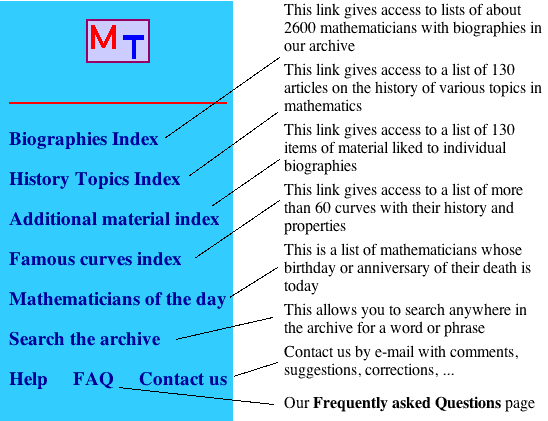## MacTutor History HelpOther links in the Main Index are to:

Birthplace Maps

Anniversaries for the year
gives lists of mathematicians who were born or died on each day of the year.

Mathematical Societies
gives information on more than 90 Mathematical societies.

Mathematical Societies, Medals, honours, etc
gives histories of mathematical societies and various honours that have
been given to Mathematicians in our archive

Timelines
let you see how the lives of mathematicians in our archive overlapped.

Chronologies
gives a chronolgy of mathematics from ancient times to the present day.

Quotations
gives quotations from many of the mathematicians in our archive.

Mathematical education
gives details of how mathematical education has developed over the centuries.

There are also links to the Edinburgh Mathematical Society,
the British Mathematical Colloquium and to
Other Web sources on the History of Mathematics.